1. 分词
2. 统计词频
3. 过滤一些语气助词，防止一些爱发嗲的，给你来个 呀 咩 碟 等
4. 通过词频获取余弦夹角来计算相似度

句子A：我/喜欢/看/电视，不/喜欢/看/电影。

句子B：我/不/喜欢/看/电视，也/不/喜欢/看/电影。

``````我，喜欢，看，电视，电影，不，也。
``````

``````句子A：我 1，喜欢 2，看 2，电视 1，电影 1，不 1，也 0。

``````

``````句子A：[1, 2, 2, 1, 1, 1, 0]

``````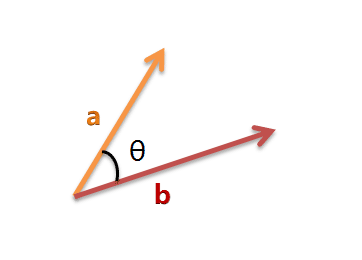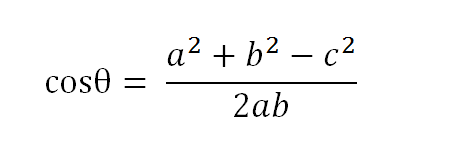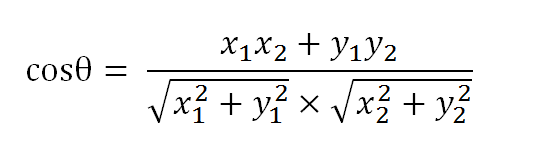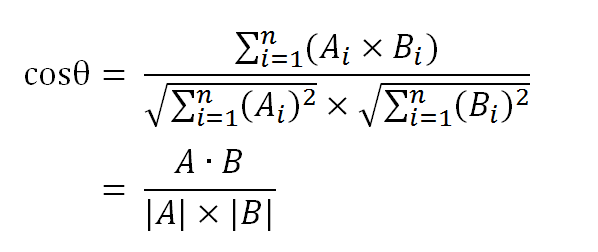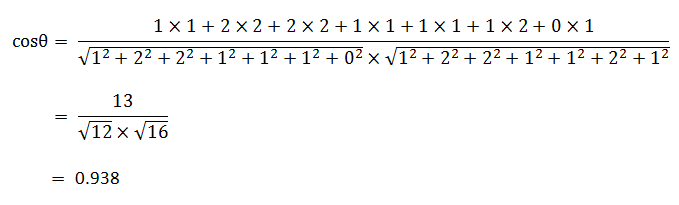``````（1）使用TF-IDF算法，找出两篇文章的关键词；
（2）每篇文章各取出若干个关键词（比如20个），合并成一个集合，计算每篇文章对于这个集合中的词的词频（为了避免文章长度的差异，可以使用相对词频）；
（3）生成两篇文章各自的词频向量；
（4）计算两个向量的余弦相似度，值越大就表示越相似。
``````

``````/*
* Use:
* \$obj = new Wd_TextSimilarity();
* echo \$obj->getSimilar(\$text1, \$text2);
*/
Class Wd_TextSimilarity {
/**
* [排除的词语]
*
* @var array
*/
private \$_excludeArr = array('的','了','和','呢','啊','哦','恩','嗯','吧');

/**
* [词语分布数组]
*
* @var array
*/
private \$_words = array();

/**
* [分词后的数组一]
*
* @var array
*/
private \$_segWrods1 = array();

/**
* [分词后的数组二]
*
* @var array
*/
private \$_segWrods2 = array();

public function __construct() {

}

/**
* [外部调用，根据待比较的两段文字获取相似度结果]
*
* @param [string] \$text1 [文本一]
* @param [string] \$text2 [文本二]
* @return [float] [相似度得分，最大值1]
*/
public function getSimilar(\$text1, \$text2) {
\$this->_segWrods1 = \$this->segment(\$text1);
\$this->_segWrods2 = \$this->segment(\$text2);

\$rate = \$this->analyse();

return \$rate;
}

/**
* [分析两段文字]
* [处理相似度]
*
* @return [float] [相似度得分]
*/
private function analyse() {
\$allwords = array();

//t1
foreach(\$this->_segWrods1 as \$word) {
if( !in_array(\$word , \$this->_excludeArr) ){
if( !array_key_exists(\$word , \$allwords) ){
\$allwords[\$word] = array(1 , 0);
}else{
\$allwords[\$word] += 1;
}
}
}

//t2
foreach(\$this->_segWrods2 as \$word){
if( !in_array(\$word , \$this->_excludeArr) ){
if( !array_key_exists(\$word , \$allwords) ){
\$allwords[\$word] = array(0 , 1);
}else{
\$allwords[\$word] += 1;
}
}
}

\$sum = \$sumT1 = \$sumT2 = 0;
foreach(\$allwords as \$word){
\$sum    += \$word * \$word;
\$sumT1  += pow(\$word, 2);
\$sumT2  += pow(\$word, 2);
}

\$rate = \$sum / (sqrt(\$sumT1 * \$sumT2));
return \$rate;
}

/**
* [分词，目前是单字分词法 (分词只是一个简单的例子，你可以使用任意的分词服务)]
*
* @param [string] \$text [待分词的文本内容]
* @return [array] [分词后的结果]
*/
private function segment(\$text) {
\$len = strlen(\$text);

if(\$len == 0) {
return array();
}

\$words = array();
for(\$i = 0; \$i < \$len; \$i++){
\$c = \$text[\$i];
\$n = ord(\$c);
if((\$n >> 7) == 0){     //0xxx xxxx, asci, single
\$words[] = \$c;
} else if((\$n >> 4) == 15){     //1111 xxxx, first in four char
if(\$i < \$len - 3){
\$words[] = \$c.\$text[\$i + 1].\$text[\$i + 2].\$text[\$i + 3];
\$i += 3;
}
} else if((\$n >> 5) == 7){  //111x xxxx, first in three char
if(\$i < \$len - 2){
\$words[] = \$c.\$text[\$i + 1].\$text[\$i + 2];
\$i += 2;
}
} else if((\$n >> 6) == 3){  //11xx xxxx, first in two char
if(\$i < \$len - 1){
\$words[] = \$c.\$text[\$i + 1];
\$i++;
}
}
}

return \$words;
}
}
``````